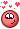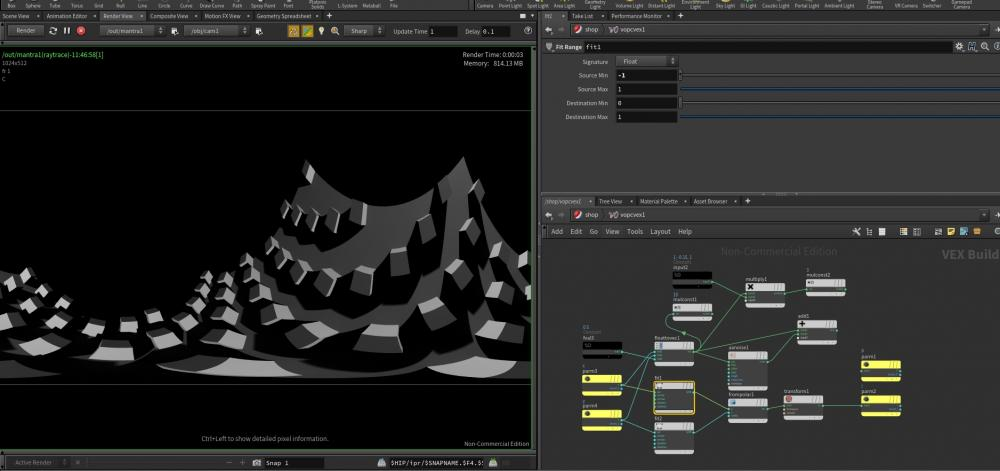## Recommended Posts

Hello!

I am struggling to get what I want from a lens shader. It is somehow a math challenge. Let's see if there is someone who can figure it out...

Simple scenario:

- Perspective camera looking towards a grid made of 4 points (corners).

- I want the camera to render the grid taking each corner as a reference to fill the frame completely with the grid (not more or less).

Details:

- The grid should be able to rotate on 3 axes and the camera should keep framing it. (So aspect ratio won't do it, since it has some perspective).

- I have managed to do it in one axis, but additional axes seem to start acting weird, the results are close but not enough. Since it is math it should be perfect!

(I does not have to be foolproof, looking towards the Z direction always and not handling more than 180-degree rotations is ok).

Code of my lens shader

```#include "math.h"

#pragma hint    x       hidden
#pragma hint    y       hidden
#pragma hint    Time    hidden
#pragma hint    dofx    hidden
#pragma hint    dofy    hidden
#pragma hint    aspect  hidden
#pragma hint    P       hidden
#pragma hint    I       hidden

cvex anamorphic_lens(

//Inputs
float x = 0; // X screen coordinate in the range -1 to 1
float y = 0; // Y screen coordinate in the range -1 to 1
float Time = 0; // Sample time
float dofx = 0; // X depth of field sample value
float dofy = 0; // Y depth of field sample value
float aspect = 1; // Image aspect ratio (x/y)

//Outputs
export vector P = 0; // Ray origin in camera space
export vector I = 0; // Ray direction in camera space
export int valid = 1; // Whether the sample is valid for measuring

//Grid corners converted to camera space
vector corner0 = 0;
vector corner3 = 0;

)
{

float xmin_bounds = corner0;
float xmax_bounds = corner3;
float ymin_bounds = corner0;
float ymax_bounds = corner3;
float zmin_bounds = corner0;
float zmax_bounds = corner3;

float new_x = fit(x, -1, 1, min(xmin_bounds, xmax_bounds), max(xmin_bounds, xmax_bounds));
float new_y = fit(y, -1, 1, min(ymin_bounds, ymax_bounds), max(ymin_bounds, ymax_bounds));

// Calculations Z axis in relation to X
float offset = min(zmin_bounds, zmax_bounds);

float relation_x = (max(zmin_bounds, zmax_bounds)-offset)/min(xmin_bounds, xmax_bounds);
if(xmin_bounds > xmax_bounds){
relation_x *= -1;
}

float relation_y = (max(zmin_bounds, zmax_bounds)-offset)/min(ymin_bounds, ymax_bounds);
if(ymin_bounds > ymax_bounds){
relation_y *= -1;
}

float z = (-relation_x * new_x)  + (-relation_y * new_y) + offset;

P = set(0, 0, 0);
I = set(new_x, new_y, z);```

Edited by Alan Monroig

##### Share on other sites

I have this in VOPCVEX..Fun ..DOnt knowjust Sharing..play with 3 methods ..## Create an account or sign in to comment

You need to be a member in order to leave a comment

## Create an account

Sign up for a new account in our community. It's easy!

Register a new account